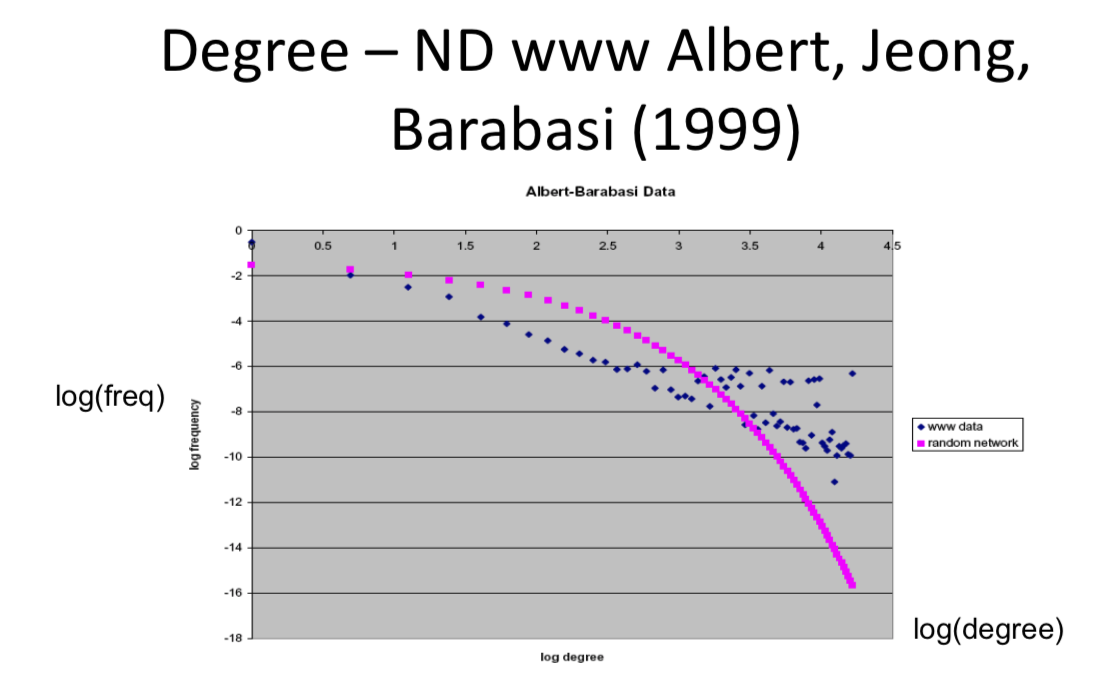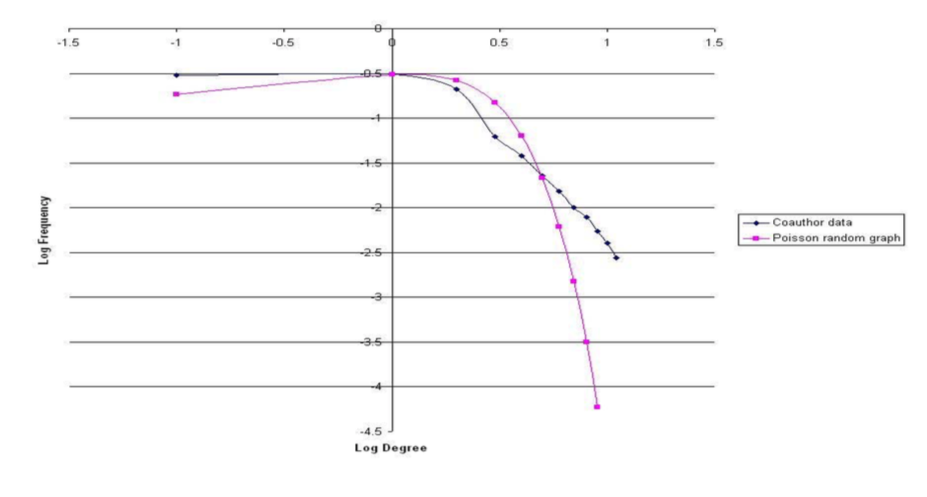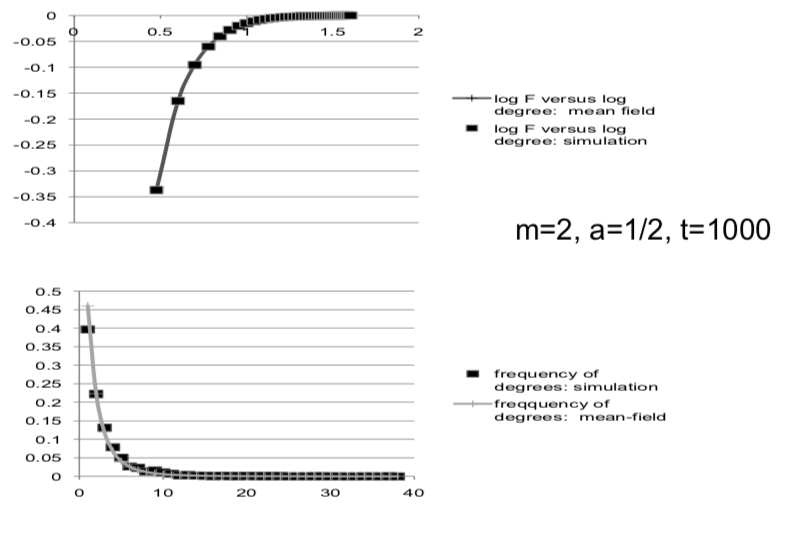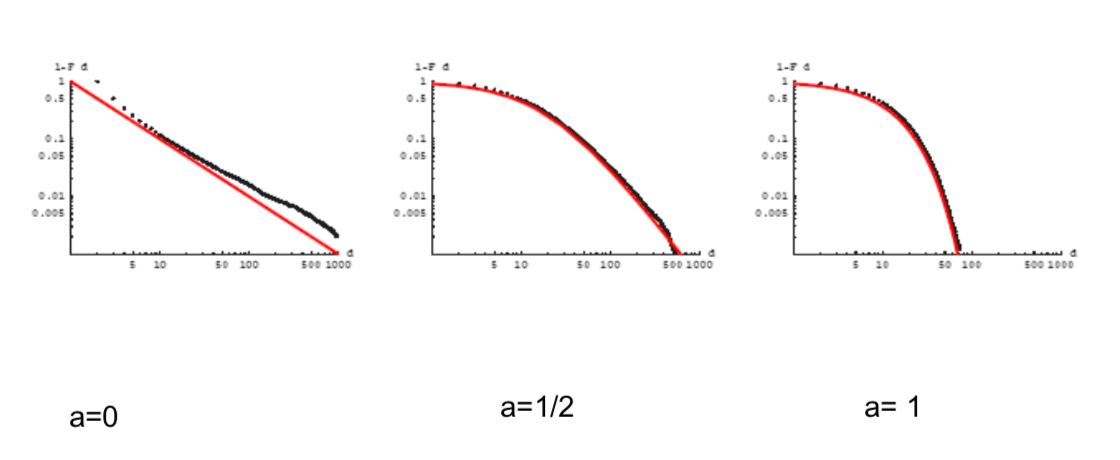# 3.4 Hybrid Models¶

A hybrid model spans between uniform at random models and the preferential attachment model

## Simple Hybrid¶

Fraction a uniformly at random, 1-a via searching neighborhoods of friends

• What we're going to is we're going to have one fraction formed uniformly at random(a) and then the rest(1-a) fraction formed via meeting friends of friends, the second part is going to look like preferential attachment. So, that will give us an explanation for how preferential attachment might actually be working.## Meeting 'Friends of Friends'¶

• Find new nodes via others: Network-based search
• New node meets am nodes uniformly at random and directs links to them
• Meets (1-a)m of their neighbors and attaches to them too
• The distribution of neighbors' nodes is not the same as the degree distribution - even with independent link formation
• A neighbor is more likely to be higher degree

## Relation to Preferential Attachment:¶

• In a network with half degree k and half degree 2k individuals:
• randomly select a link and then a node on one end of it - 2/3 chance that it has degree 2k, 1/3 chance that it has degree k
• Suppose in a network with half degree k individuals and half degree 3k individuals, you randomly select a link and then a node on one end of it. What is the probability that the node found has degree 3K?
• Following the procedure described, the chance of finding a node with degree 3k is 3k/k = 3 times of the chance of finding a node with degree k. Hence, the probability of finding a node with degree 3k is 3/(3+1) = 0.75
• Randomly find a node, randomly pick one of the nodes it attached to(that's the sort of finding of friends of friends bit), chance of finding some node via the second part of this procedure is proportional to its degree: find it if find one of its neighbors...

## Simple Hybrid¶

• Fraction a uniformly at random, 1-a via preferential attachment: $$dd_i(t)/dt = am/t + (1 - a)d_i(t)/2t$$ and starting condition: $d_i(i) = m$, and the solution of this differential equation is:

$$d_i(t) = (m + 2am/(1-a))(t/i)^{(1-a)/2} - 2am/(1-a)$$

What this does is tell us what an expected degree is, at any point in time for a given node.

## Degree distribution¶

Nodes that have expected degree less than d at some time t are those i such that

$(m + xam)(t/i)^{1/x} - xam <d$ where $x = 2/(1-a)$

ciritical i is such that

$i/t = [(m + xam)/(d + xam)]^x$ where $x = 2/(1-a)$

$F(d) = (t - i)/t$

$F(d) = 1 - ((m + amx) / (d + amx))^x$ where $x = 2/(1-a)$

## Spans Extremes¶

$F(d) = 1 - ((m + amx) / (d + amx))^x$ where $x = 2/(1-a)$

• As a near 1, so that all of the links are being formed uniformly at random, then this is nearly a negative exponential distribution.
• As a near 0, then the x just becames a 2, and we're going to get our 1 minus m over d raised to the 2 power the differentiative, the density function, this look exactly like preferential attachment

# Simulate to check¶# Degree Distributions as vary the random/search parameter¶